# Operators in Python

19. Aug 2019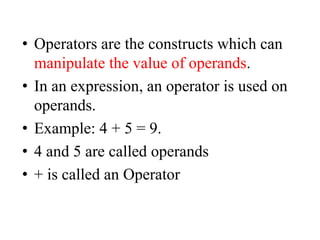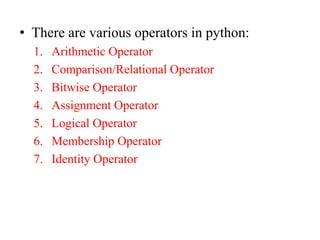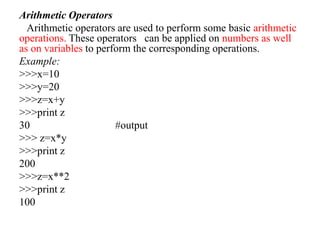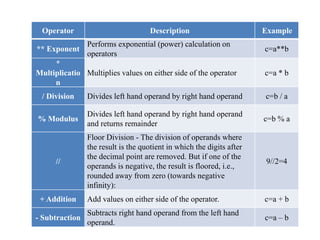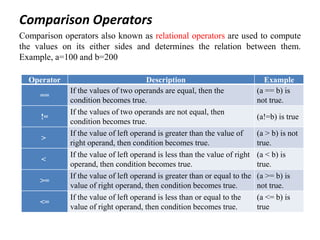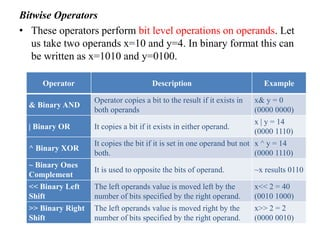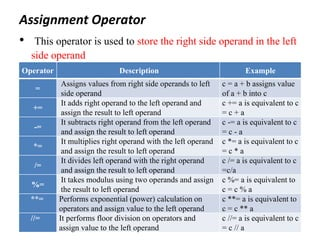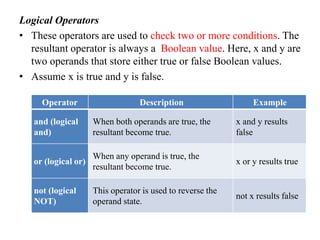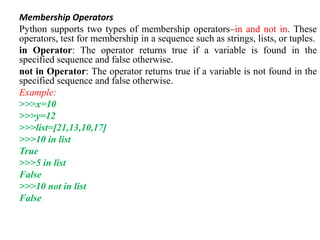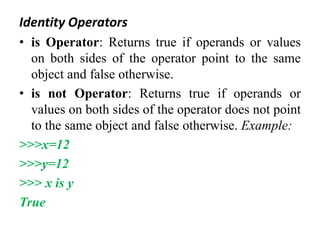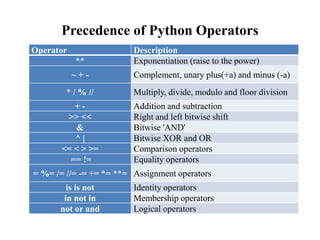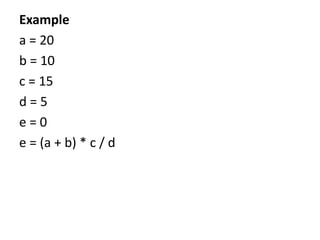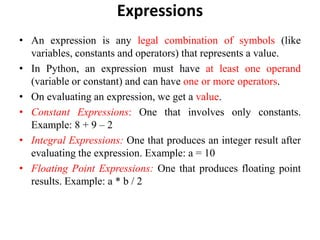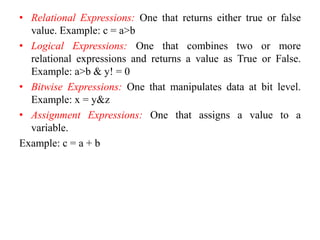1 von 15

### Operators in Python

• 1. Operators in Python V.Anusuya Assistant Professor(SG)/CSE Ramco Institute of Technology
• 2. • Operators are the constructs which can manipulate the value of operands. • In an expression, an operator is used on operands. • Example: 4 + 5 = 9. • 4 and 5 are called operands • + is called an Operator
• 3. • There are various operators in python: 1. Arithmetic Operator 2. Comparison/Relational Operator 3. Bitwise Operator 4. Assignment Operator 5. Logical Operator 6. Membership Operator 7. Identity Operator
• 4. Arithmetic Operators Arithmetic operators are used to perform some basic arithmetic operations. These operators can be applied on numbers as well as on variables to perform the corresponding operations. Example: >>>x=10 >>>y=20 >>>z=x+y >>>print z 30 #output >>> z=x*y >>>print z 200 >>>z=x**2 >>>print z 100
• 5. Operator Description Example ** Exponent Performs exponential (power) calculation on operators c=a**b * Multiplicatio n Multiplies values on either side of the operator c=a * b / Division Divides left hand operand by right hand operand c=b / a % Modulus Divides left hand operand by right hand operand and returns remainder c=b % a // Floor Division - The division of operands where the result is the quotient in which the digits after the decimal point are removed. But if one of the operands is negative, the result is floored, i.e., rounded away from zero (towards negative infinity): 9//2=4 + Addition Add values on either side of the operator. c=a + b - Subtraction Subtracts right hand operand from the left hand operand. c=a – b
• 6. Comparison Operators Comparison operators also known as relational operators are used to compute the values on its either sides and determines the relation between them. Example, a=100 and b=200 Operator Description Example == If the values of two operands are equal, then the condition becomes true. (a == b) is not true. != If the values of two operands are not equal, then condition becomes true. (a!=b) is true > If the value of left operand is greater than the value of right operand, then condition becomes true. (a > b) is not true. < If the value of left operand is less than the value of right operand, then condition becomes true. (a < b) is true. >= If the value of left operand is greater than or equal to the value of right operand, then condition becomes true. (a >= b) is not true. <= If the value of left operand is less than or equal to the value of right operand, then condition becomes true. (a <= b) is true
• 7. Bitwise Operators • These operators perform bit level operations on operands. Let us take two operands x=10 and y=4. In binary format this can be written as x=1010 and y=0100. Operator Description Example & Binary AND Operator copies a bit to the result if it exists in both operands x& y = 0 (0000 0000) | Binary OR It copies a bit if it exists in either operand. x | y = 14 (0000 1110) ^ Binary XOR It copies the bit if it is set in one operand but not both. x ^ y = 14 (0000 1110) ~ Binary Ones Complement It is used to opposite the bits of operand. ~x results 0110 << Binary Left Shift The left operands value is moved left by the number of bits specified by the right operand. x<< 2 = 40 (0010 1000) >> Binary Right Shift The left operands value is moved right by the number of bits specified by the right operand. x>> 2 = 2 (0000 0010)
• 8. Assignment Operator • This operator is used to store the right side operand in the left side operand Operator Description Example = Assigns values from right side operands to left side operand c = a + b assigns value of a + b into c += It adds right operand to the left operand and assign the result to left operand c += a is equivalent to c = c + a -= It subtracts right operand from the left operand and assign the result to left operand c -= a is equivalent to c = c - a *= It multiplies right operand with the left operand and assign the result to left operand c *= a is equivalent to c = c * a /= It divides left operand with the right operand and assign the result to left operand c /= a is equivalent to c =c/a %= It takes modulus using two operands and assign the result to left operand c %= a is equivalent to c = c % a **= Performs exponential (power) calculation on operators and assign value to the left operand c **= a is equivalent to c = c ** a //= It performs floor division on operators and assign value to the left operand c //= a is equivalent to c = c // a
• 9. Logical Operators • These operators are used to check two or more conditions. The resultant operator is always a Boolean value. Here, x and y are two operands that store either true or false Boolean values. • Assume x is true and y is false. Operator Description Example and (logical and) When both operands are true, the resultant become true. x and y results false or (logical or) When any operand is true, the resultant become true. x or y results true not (logical NOT) This operator is used to reverse the operand state. not x results false
• 10. Membership Operators Python supports two types of membership operators–in and not in. These operators, test for membership in a sequence such as strings, lists, or tuples. in Operator: The operator returns true if a variable is found in the specified sequence and false otherwise. not in Operator: The operator returns true if a variable is not found in the specified sequence and false otherwise. Example: >>>x=10 >>>y=12 >>>list=[21,13,10,17] >>>10 in list True >>>5 in list False >>>10 not in list False
• 11. Identity Operators • is Operator: Returns true if operands or values on both sides of the operator point to the same object and false otherwise. • is not Operator: Returns true if operands or values on both sides of the operator does not point to the same object and false otherwise. Example: >>>x=12 >>>y=12 >>> x is y True
• 12. Precedence of Python Operators Operator Description ** Exponentiation (raise to the power) ~ + - Complement, unary plus(+a) and minus (-a) * / % // Multiply, divide, modulo and floor division + - Addition and subtraction >> << Right and left bitwise shift & Bitwise 'AND' ^ | Bitwise XOR and OR <= < > >= Comparison operators == != Equality operators = %= /= //= -= += *= **= Assignment operators is is not Identity operators in not in Membership operators not or and Logical operators
• 13. Example a = 20 b = 10 c = 15 d = 5 e = 0 e = (a + b) * c / d
• 14. Expressions • An expression is any legal combination of symbols (like variables, constants and operators) that represents a value. • In Python, an expression must have at least one operand (variable or constant) and can have one or more operators. • On evaluating an expression, we get a value. • Constant Expressions: One that involves only constants. Example: 8 + 9 – 2 • Integral Expressions: One that produces an integer result after evaluating the expression. Example: a = 10 • Floating Point Expressions: One that produces floating point results. Example: a * b / 2
• 15. • Relational Expressions: One that returns either true or false value. Example: c = a>b • Logical Expressions: One that combines two or more relational expressions and returns a value as True or False. Example: a>b & y! = 0 • Bitwise Expressions: One that manipulates data at bit level. Example: x = y&z • Assignment Expressions: One that assigns a value to a variable. Example: c = a + b